# Data simulation and propensity score estimation

In this post, I will play around with simulated data. The things I’ll be doing:

• Simulating my own dataset with null associations between two different exposures (x1 and x2) and outcomes y1 and y2 for each of exposures (4 exposure-outcome pairs)
• Computing propensity scores (PS) for each exposure, trimming non-overlapping areas of PS distribution between exposed and unexposed
• Running several logistic regression models
• Crude
• Adjusted with standardized mortality ratio (SMR) weighting using PS
• Calculating how biased the the estimates are compared with the true (null) effect

## Data simulation

First, I simulate the data for 10 confounders c1-c10, 2 exposures x1 and x2 (with 7% and 20% prevalences, respectively), 2 outcomes (y1 and y2), two exposure predictors c11-c12, and 2 predictors of the outcome c13-c14. The post is inspired by Desai et al. 2017 paper.

set.seed(08032021)

# simulate data size
n = 2e4

# create 10 confounders
c1 <- rbinom(n, size = 1, prob = 0.052)
c2 <- rbinom(n, size = 1, prob = 0.03)
c3 <- rbinom(n, size = 1, prob = 0.042)
c4 <- rbinom(n, size = 1, prob = 0.025)
c5 <- rbinom(n, size = 1, prob = 0.038)
c6 <- rbinom(n, size = 1, prob = 0.053)
c7 <- rbinom(n, size = 1, prob = 0.077)
c8 <- rbinom(n, size = 1, prob = 0.017)
c9 <- rbinom(n, size = 1, prob = 0.043)
c10 <- rnorm(n = n, mean = 32, sd = 7)

# treatment determinant only
c11 <- rbinom(n, size = 1, prob = 0.2)
c12 <- rbinom(n, size = 1, prob = 0.12)

# outcome determinant only
c13 <- rbinom(n, size = 1, prob = 0.27)
c14 <- rbinom(n, size = 1, prob = 0.09)

# A binary exposure variable
a1 <- -5.05
a2 <- -4.18

# Predict the probability of the exposures x1 and x2 using 10 confounders and two exposure determinants (c1–c12)

x1 <- rbinom(n, size = 1, prob = locfit::expit(a1 + 0.34*c1 + 0.29*c2 + 2.96*c3 + 0.4*c4 + 0.13*c5 + 1.51*c6 + 1.69*c7 + 1.10*c8 + 0.23*c9 + 0.05*c10 + 0.4*c11 + 0.3*c12))

x2 <- rbinom(n, size = 1, prob = locfit::expit(a2 + 0.10*c1 + 0.30*c2 + 1.05*c3 + 0.45*c4 + 0.24*c5 + 0.13*c6 + 2.05*c7 + 0.19*c8 + 0.3*c9 + 0.07*c10 + 0.6*c11 + 0.1*c12))

# A binary outcome variable is simulated using c1-c10 confounders and outcome predictors. The null exposure effect: x variables are not used to simulate the outcomes

b1 <- -3.15
b2 <- -2.1

y1 <- rbinom(n = n, size = 1, prob = locfit::expit(b1 + 0.12*c1 + 0.06*c2 + 0.09*c3 + 0.10*c4 + 0.15*c5 + 0.3*c6 + 0.11*c7 + 0.1786*c8 + 0.5924*c9 + 0.002*c10 + 0.4*c13 + 0.5*c14))

y2 <- rbinom(n = n, size = 1, prob = locfit::expit(b2 + 0.24*c1 + 0.07*c2 + 0.09*c3 + 0.13*c4 + 0.52*c5 + 0.26*c6 + 0.17*c7 + 0.86*c8 + 0.29*c9 + 0.01*c10 + 0.009*c13 + 0.1*c14))

# assemble the dataset
data <- data_frame(c1, c2, c3, c4, c5, c6, c7, c8, c9, c10, c11, c12, c13, c14, x1, x2, y1, y2)

## Warning: data_frame() was deprecated in tibble 1.1.0.
## Please use tibble() instead.

skimr::skim(data)


Table: Table 1: Data summary

Name data
Number of rows 20000
Number of columns 18
_______________________
Column type frequency:
numeric 18
________________________
Group variables None

Variable type: numeric

skim_variable n_missing complete_rate mean sd p0 p25 p50 p75 p100 hist
c1 0 1 0.06 0.23 0.00 0.00 0.00 0.00 1.00 ▇▁▁▁▁
c2 0 1 0.03 0.17 0.00 0.00 0.00 0.00 1.00 ▇▁▁▁▁
c3 0 1 0.04 0.20 0.00 0.00 0.00 0.00 1.00 ▇▁▁▁▁
c4 0 1 0.03 0.16 0.00 0.00 0.00 0.00 1.00 ▇▁▁▁▁
c5 0 1 0.04 0.20 0.00 0.00 0.00 0.00 1.00 ▇▁▁▁▁
c6 0 1 0.05 0.22 0.00 0.00 0.00 0.00 1.00 ▇▁▁▁▁
c7 0 1 0.08 0.26 0.00 0.00 0.00 0.00 1.00 ▇▁▁▁▁
c8 0 1 0.02 0.13 0.00 0.00 0.00 0.00 1.00 ▇▁▁▁▁
c9 0 1 0.04 0.20 0.00 0.00 0.00 0.00 1.00 ▇▁▁▁▁
c10 0 1 32.04 6.97 5.42 27.34 32.07 36.81 59.52 ▁▃▇▃▁
c11 0 1 0.20 0.40 0.00 0.00 0.00 0.00 1.00 ▇▁▁▁▂
c12 0 1 0.12 0.32 0.00 0.00 0.00 0.00 1.00 ▇▁▁▁▁
c13 0 1 0.26 0.44 0.00 0.00 0.00 1.00 1.00 ▇▁▁▁▃
c14 0 1 0.09 0.28 0.00 0.00 0.00 0.00 1.00 ▇▁▁▁▁
x1 0 1 0.08 0.26 0.00 0.00 0.00 0.00 1.00 ▇▁▁▁▁
x2 0 1 0.20 0.40 0.00 0.00 0.00 0.00 1.00 ▇▁▁▁▂
y1 0 1 0.06 0.23 0.00 0.00 0.00 0.00 1.00 ▇▁▁▁▁
y2 0 1 0.15 0.36 0.00 0.00 0.00 0.00 1.00 ▇▁▁▁▂

Creating two separate datasets: one for each exposure

# mutate confounders to factor variables for easier readability by humans :)
data %<>%
mutate_at(c("c1", "c2", "c3", "c4", "c5", "c6", "c7", "c8", "c9", "c11", "c12", "c13", "c14", "y1", "y2", "x1", "x2"), ~ as_factor(.)
)

data1 <- data %>% select(-c(x2), x = x1)
data2 <- data %>% select(-c(x1), x = x2)


Checking the variables distributions

data1 %>%
group_by(x) %>%
furniture::table1(.) %>%
as_tibble() %>%
ungroup() %>%
rename("x" = 1, "x=0" = 2, "x=1" = 3) %>%
mutate_all(~stringr::str_trim(., side = "both")) %>%
filter(x != "0") %>%
kableExtra::kable()

## Using dplyr::group_by() groups: x

x x=0 x=1
n = 18499 n = 1501
c1
1 1001 (5.4%) 102 (6.8%)
c2
1 536 (2.9%) 45 (3%)
c3
1 428 (2.3%) 384 (25.6%)
c4
1 455 (2.5%) 49 (3.3%)
c5
1 743 (4%) 66 (4.4%)
c6
1 850 (4.6%) 208 (13.9%)
c7
1 1174 (6.3%) 344 (22.9%)
c8
1 273 (1.5%) 56 (3.7%)
c9
1 781 (4.2%) 74 (4.9%)
c10
31.9 (7.0) 33.8 (6.8)
c11
1 3548 (19.2%) 407 (27.1%)
c12
1 2176 (11.8%) 219 (14.6%)
c13
1 4882 (26.4%) 416 (27.7%)
c14
1 1600 (8.6%) 148 (9.9%)
y1
1 1041 (5.6%) 86 (5.7%)
y2
1 2850 (15.4%) 242 (16.1%)
data2 %>%
group_by(x) %>%
furniture::table1(.) %>%
as_tibble() %>%
ungroup() %>%
rename("x" = 1, "x=0" = 2, "x=1" = 3) %>%
mutate_all(~stringr::str_trim(., side = "both"))%>%
filter(x != "0") %>%
kableExtra::kable()

## Using dplyr::group_by() groups: x

x x=0 x=1
n = 15973 n = 4027
c1
1 859 (5.4%) 244 (6.1%)
c2
1 437 (2.7%) 144 (3.6%)
c3
1 521 (3.3%) 291 (7.2%)
c4
1 355 (2.2%) 149 (3.7%)
c5
1 610 (3.8%) 199 (4.9%)
c6
1 835 (5.2%) 223 (5.5%)
c7
1 620 (3.9%) 898 (22.3%)
c8
1 262 (1.6%) 67 (1.7%)
c9
1 634 (4%) 221 (5.5%)
c10
31.4 (6.9) 34.4 (6.8)
c11
1 2860 (17.9%) 1095 (27.2%)
c12
1 1872 (11.7%) 523 (13%)
c13
1 4216 (26.4%) 1082 (26.9%)
c14
1 1393 (8.7%) 355 (8.8%)
y1
1 876 (5.5%) 251 (6.2%)
y2
1 2436 (15.3%) 656 (16.3%)

Making a nested dataframe to run models for each dataset in the nested dataframe.

# make a nested dataset

nested <- enframe(x = list(data1, data2), name = "dataset") %>%
rename(data = "value")

nested %<>% mutate(
dataset = c("Pr(x) = 0.07", "Pr(x) = 0.20"),
prevalence_exposure = c(0.07, 0.20),
p_x_glm_model = map(data, ~ glm(data = .x, family = binomial(link = "logit"), formula = x ~ 1)),
p_x = map(p_x_glm_model, ~predict(.x, type = "response")),
data = map2(data, p_x, ~mutate(.x,
p_x = .y)))


I run crude analyses for the association between x and y1 & x and y2 for each different x; then I compute 95% confidence intervals (CIs) using bootstrap for each of the crude analyses.

# crude analyses
set.seed(19052020)

# simple functions for crude logistic regressions of x on y
fit_crude_y1 <- function(split) {
glm(data = split, family = binomial(link = "logit"), formula = y1 ~ x)
}

fit_crude_y2 <- function(split) {
glm(data = split, family = binomial(link = "logit"), formula = y2 ~ x)
}

# run the bootstraps and the crude models
nested %<>% mutate(

boots = map(data, ~rsample::bootstraps(.x, times = 1e3, apparent = TRUE)),

boot_models_y1 = map(boots, ~mutate(.x,
model_y1 = map(splits, ~fit_crude_y1(split = .x)),
coef_info = map(model_y1, ~broom::tidy(.x, exponentiate = T)))
),

boot_models_y2 = map(boots, ~mutate(.x,
model_y2 = map(splits, ~fit_crude_y2(split = .x)),
coef_info = map(model_y2, ~broom::tidy(.x, exponentiate = T)))
),
# extract coefficients and create 95% confidence intervals using percentile method
boot_coefs_y1 = map(boot_models_y1, ~unnest(.x, coef_info)),
boot_coefs_y2 = map(boot_models_y2, ~unnest(.x, coef_info)),
percentile_intervals_y1 = map(boot_models_y1, ~int_pctl(.x, coef_info)),
percentile_intervals_y2 = map(boot_models_y2, ~int_pctl(.x, coef_info))
)


I extract the results of the crude analyses for two datasets capturing two different exposures and two different outcomes for each exposure.

results_crude <- nested %>%
select(dataset, prevalence_exposure, percentile_intervals_y1, percentile_intervals_y2) %>%
pivot_longer(cols = c(3, 4), names_to = "outcome", values_to = "percentile_intervals") %>%
unnest_legacy() %>%
mutate(outcome = str_extract(outcome, pattern = "y\\d+$")) %>% filter(term == "x1") %>% # pct bias on odds ratio (OR) scale # confidence limit ration (CLR) mutate( pct_bias = (.estimate - 1) * 100, clr = .upper/.lower )  I create a color palette from a picture I saw online. Thanks to colorfindr package for making this possible. # make a palette palette_ps <- colorfindr::get_colors("http://wisetoast.com/wp-content/uploads/2015/10/The-Persistence-of-Memory-salvador-deli-painting.jpg") %>% colorfindr::make_palette(n = 10)  ## Warning: Quick-TRANSfer stage steps exceeded maximum (= 23600600)I plot the estimates and bootstrapped 95% CIs for the sets of two exposures and outcomes y1 and y2 to visually assess the results. I know that in simulated data there is null true effect of both exposures on the outcomes y1 and y2. I suspect that the results of the crude analyses would be biased. p1 <- results_crude %>% ggplot(aes(x = as_factor(prevalence_exposure), y = .estimate, color = outcome, fill = outcome, shape = outcome, group = outcome)) + geom_hline(yintercept = 1, color = "#617E7C") + geom_errorbar(aes(ymin=.lower, ymax = .upper), width = 0.2, position = position_dodge(width = 0.3)) + geom_point(position = position_dodge(width = 0.3)) + coord_flip() + theme_light() + scale_color_manual(values = palette_ps[c(10, 9)]) + scale_fill_manual(values = palette_ps[c(10, 9)]) + labs(title = "Crude associations", x = "Exposure prevalence", y = "OR") + guides(fill=guide_legend(title = "Outcome"), color = guide_legend(title = "Outcome"), shape=guide_legend(title = "Outcome")) p2 <- results_crude %>% ggplot(aes(x = as_factor(prevalence_exposure), y = pct_bias, color = outcome, fill = outcome, shape = outcome, group = outcome)) + scale_y_continuous(limits = c(-20, 20), breaks = seq(-20, 20, 5)) + geom_hline(yintercept = 0, color = "#617E7C") + geom_point() + theme_light() + coord_flip() + scale_color_manual(values = palette_ps[c(10, 9)]) + scale_fill_manual(values = palette_ps[c(10, 9)]) + labs(title = "Crude associations", x = "Exposure prevalence", y = "% bias") + guides(fill=guide_legend(title = "Outcome"), color = guide_legend(title = "Outcome"), shape = guide_legend(title = "Outcome")) p1 / p2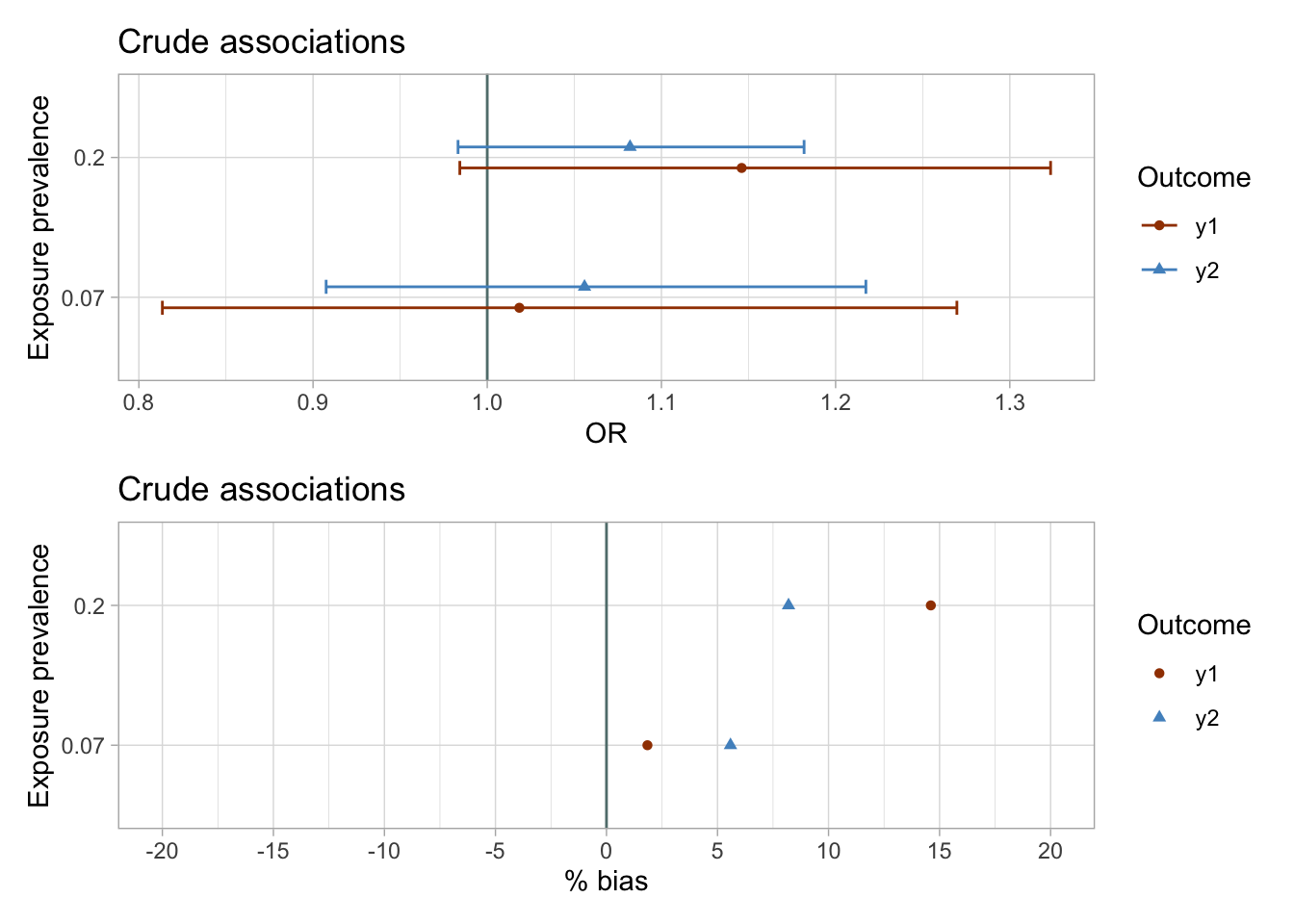The results of the unadjusted analyses are imprecise and suggest weak associations between exposures and the outcomes. ## Propensity score (PS) modeling # the 10 confounders, c1–c10, & the predictors of the outcome are included in the propensity score models ps_model <- function(data) glm(data = data, family = binomial(link = "logit"), formula = x ~ c1 + c2 + c3 + c4 + c5 + c6 + c7 + c8 + c9 + c10 + c13 + c14) nested %<>% mutate( # fit ps models in each dataset ps_fit = map(data, ~ ps_model(.x)), # tidy ps fit ps_fit_tidy = map(ps_fit, ~broom::tidy(., exponentiate = TRUE, conf.int = TRUE)), # compute predicted probability of exposure in each dataset data = map2(ps_fit, data, ~mutate(.y, p_xc = predict(.x, type = "response"))) ) # PS viz title <- list("Pr(x) = 0.07", "Pr(x) = 0.20") nested %<>% mutate( viz_density = map2(data, title, ~ggplot(data = .x, aes(x = p_xc, group = x, color = x, fill = x)) + geom_density(alpha = 0.4) + theme_light() + labs(title = paste0(.y, ", n = ", nrow(.x))) + scale_color_manual(values = palette_ps[c(10, 9)]) + scale_fill_manual(values = palette_ps[c(10, 9)]) ), viz_histo = map2(data, title, ~ggplot(data = .x, aes(x = p_xc, group = x, color = x, fill = x)) + geom_histogram(alpha = 0.6, colour = "grey", bins = 100) + theme_light() + labs(title = paste0(.y, ", n = ", nrow(.x))) + scale_color_manual(values = palette_ps[c(10, 9)]) + scale_fill_manual(values = palette_ps[c(10, 9)]) ) )  ### PS density plot and histogram I plot each PS as a density plot and as a histogram separately for exposed and unexposed. nested$viz_density

## []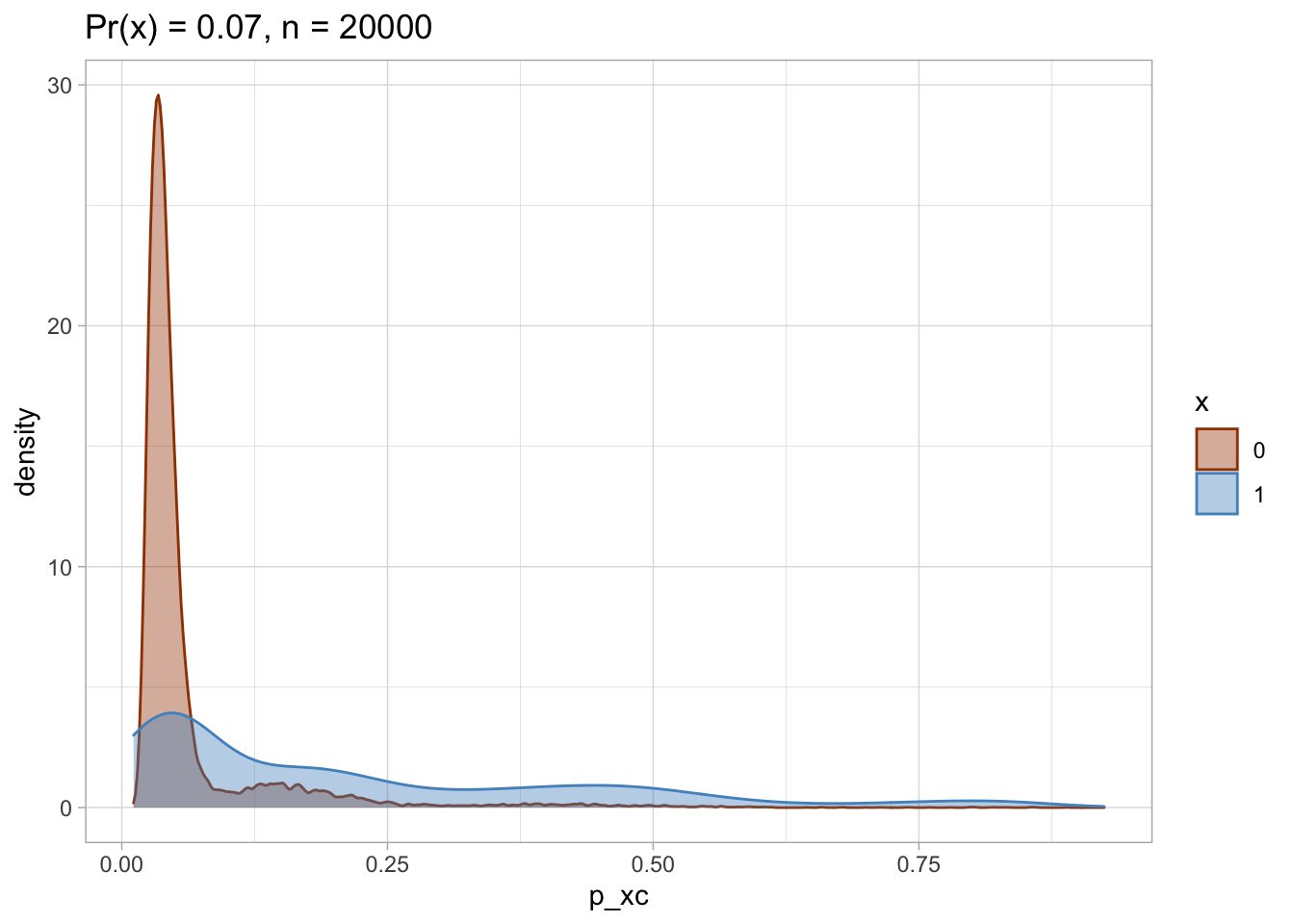##
## []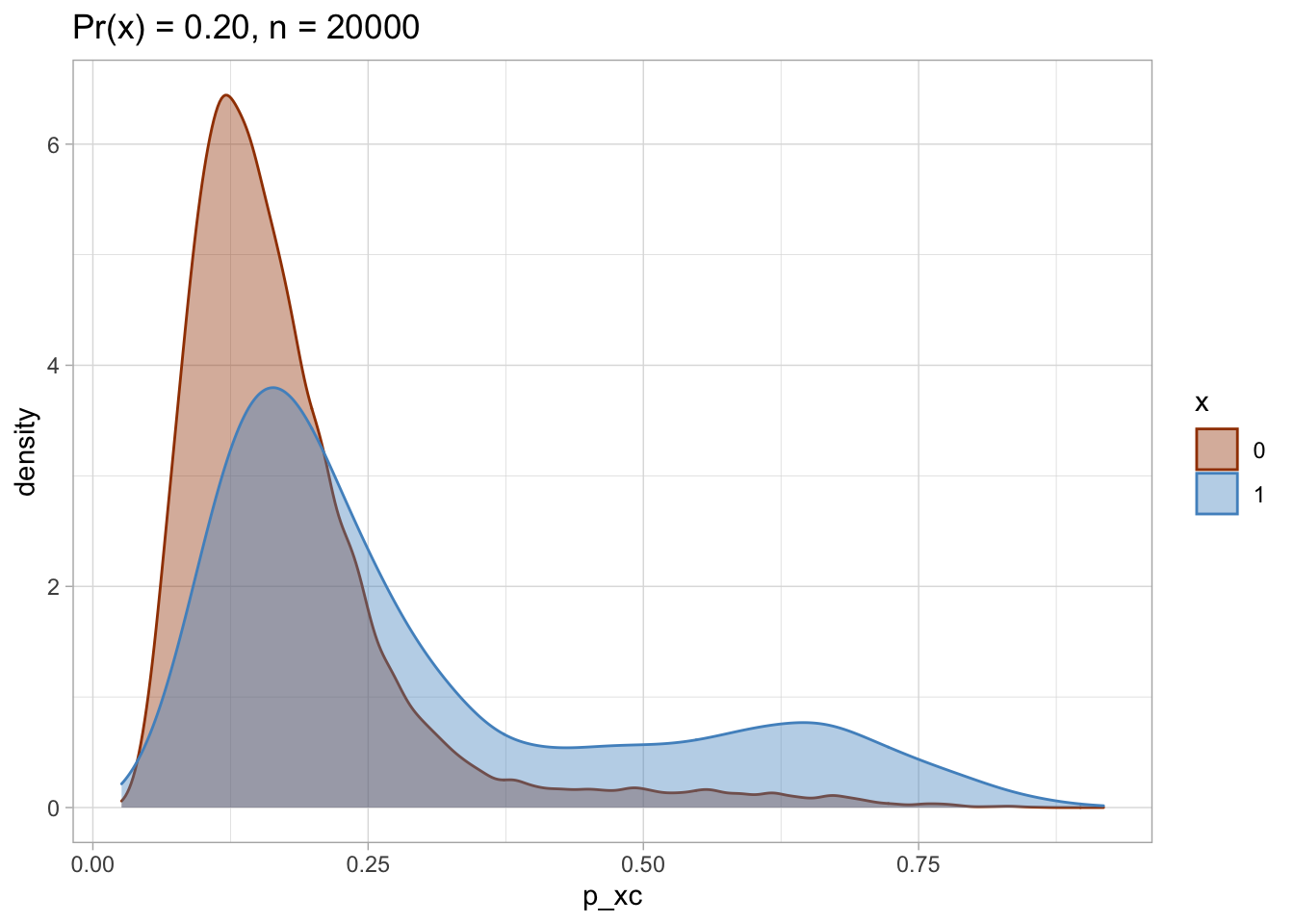nested$viz_histo  ## []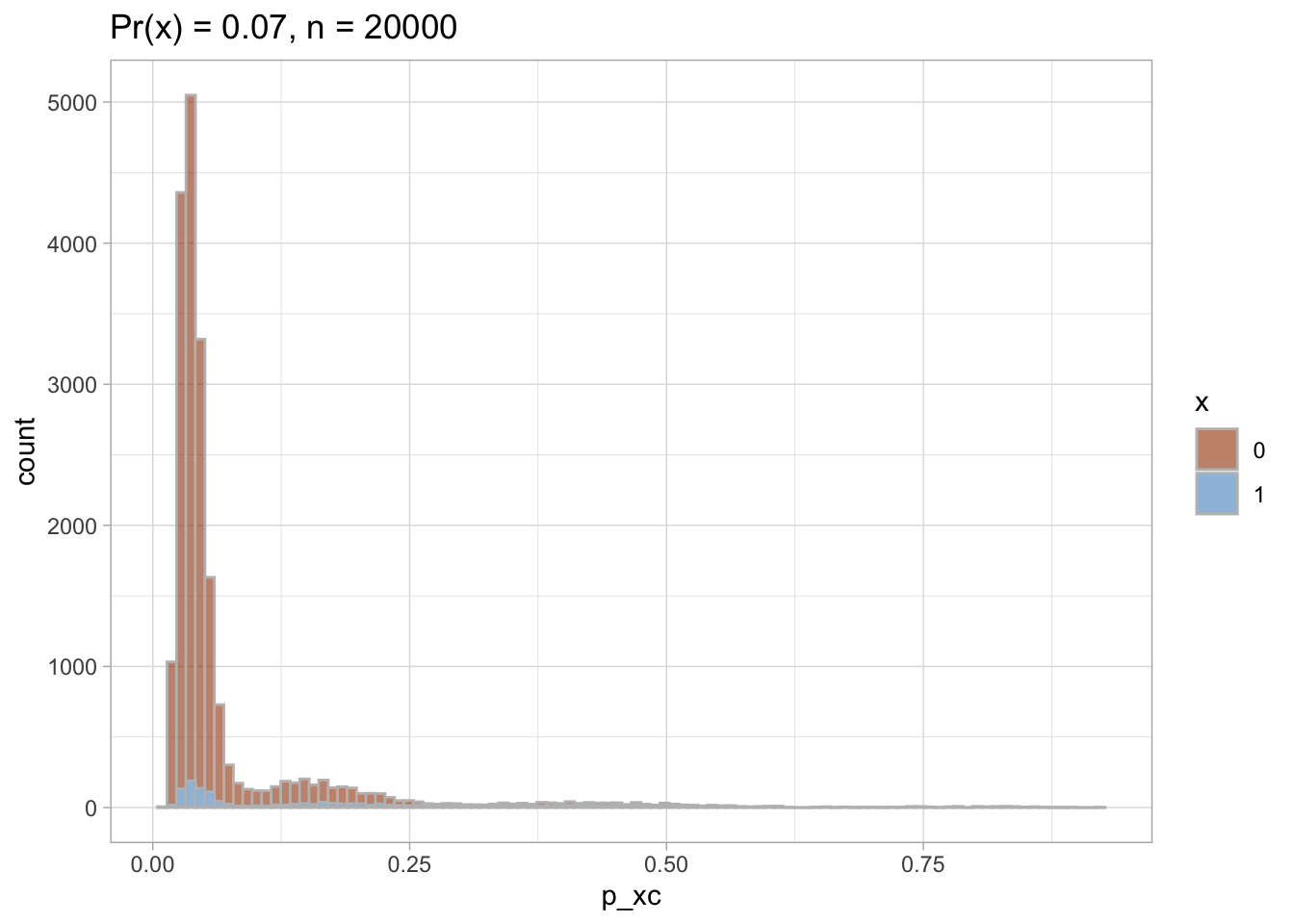## ## []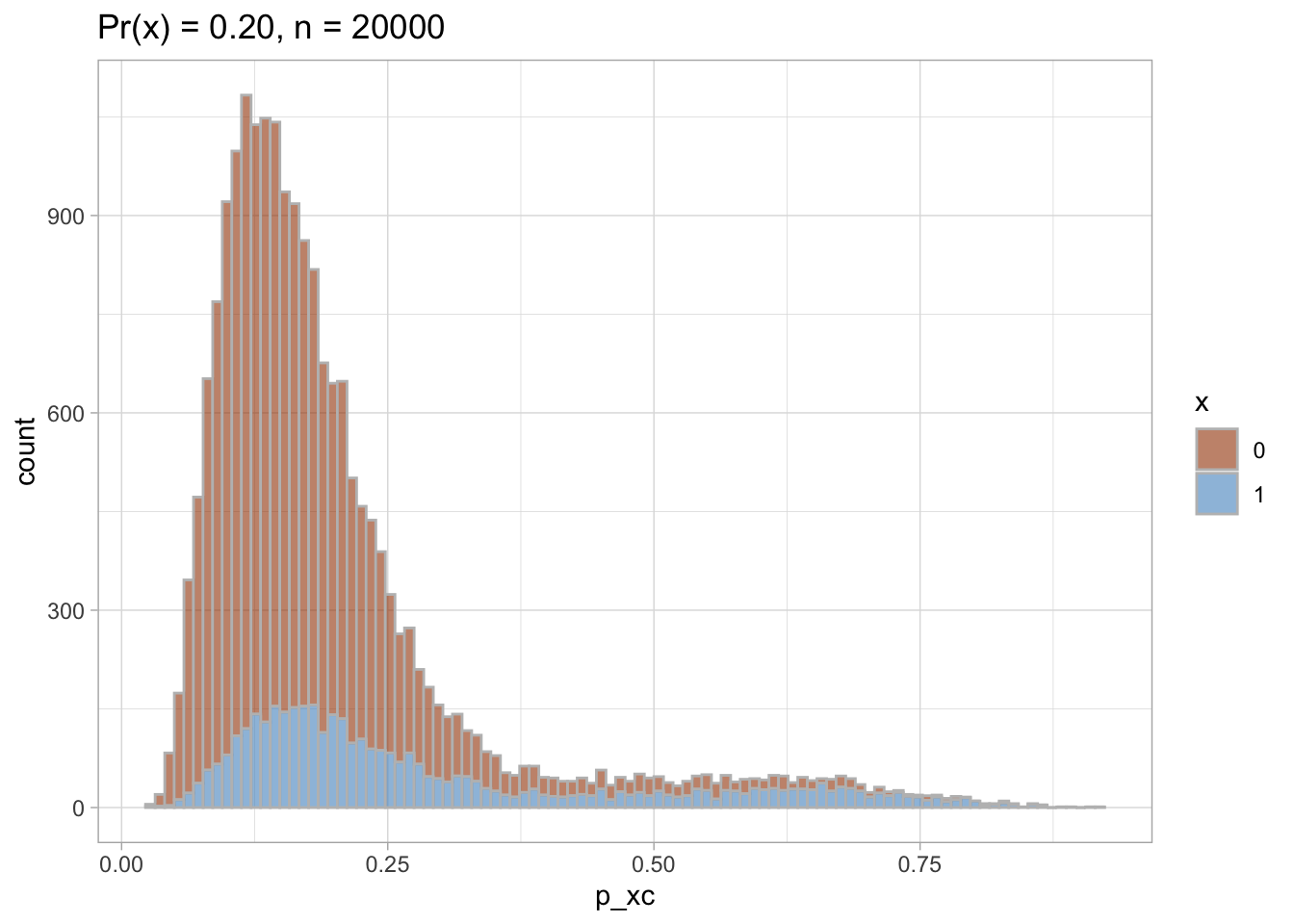### PS trimming I trim the non-overlapping areas of PS distribution in each of the datasets. # trim areas of PS non-overlap nested %<>% mutate( data_trimmed = map(data, ~mutate(.x, keep_p_xc = case_when( (p_xc >= min(p_xc[.x$x == 1])) & (p_xc <= max(p_xc[.x$x == 0])) ~ 1, T ~ 0 )) %>% filter(keep_p_xc == 1)) ) nested %<>% mutate( viz_density_trimmed = map2(data_trimmed, title, ~ggplot(data = .x, aes(x = p_xc, group = x, color = x, fill = x)) + geom_density(alpha = 0.4) + theme_light() + labs(title = paste0(.y, ", n = ", nrow(.x))) + scale_color_manual(values = palette_ps[c(10, 9)]) + scale_fill_manual(values = palette_ps[c(10, 9)]) ), viz_histo_trimmed = map2(data_trimmed, title, ~ggplot(data = .x, aes(x = p_xc, group = x, color = x, fill = x)) + geom_histogram(alpha = 0.6, colour = "grey", bins = 100) + theme_light() + labs(title = paste0(.y, ", n = ", nrow(.x))) + scale_color_manual(values = palette_ps[c(10, 9)]) + scale_fill_manual(values = palette_ps[c(10, 9)]) ) )  ### Trimmed PS density plot and histogram Let’s plot the trimmed PS densities and histograms. nested$viz_density_trimmed

## []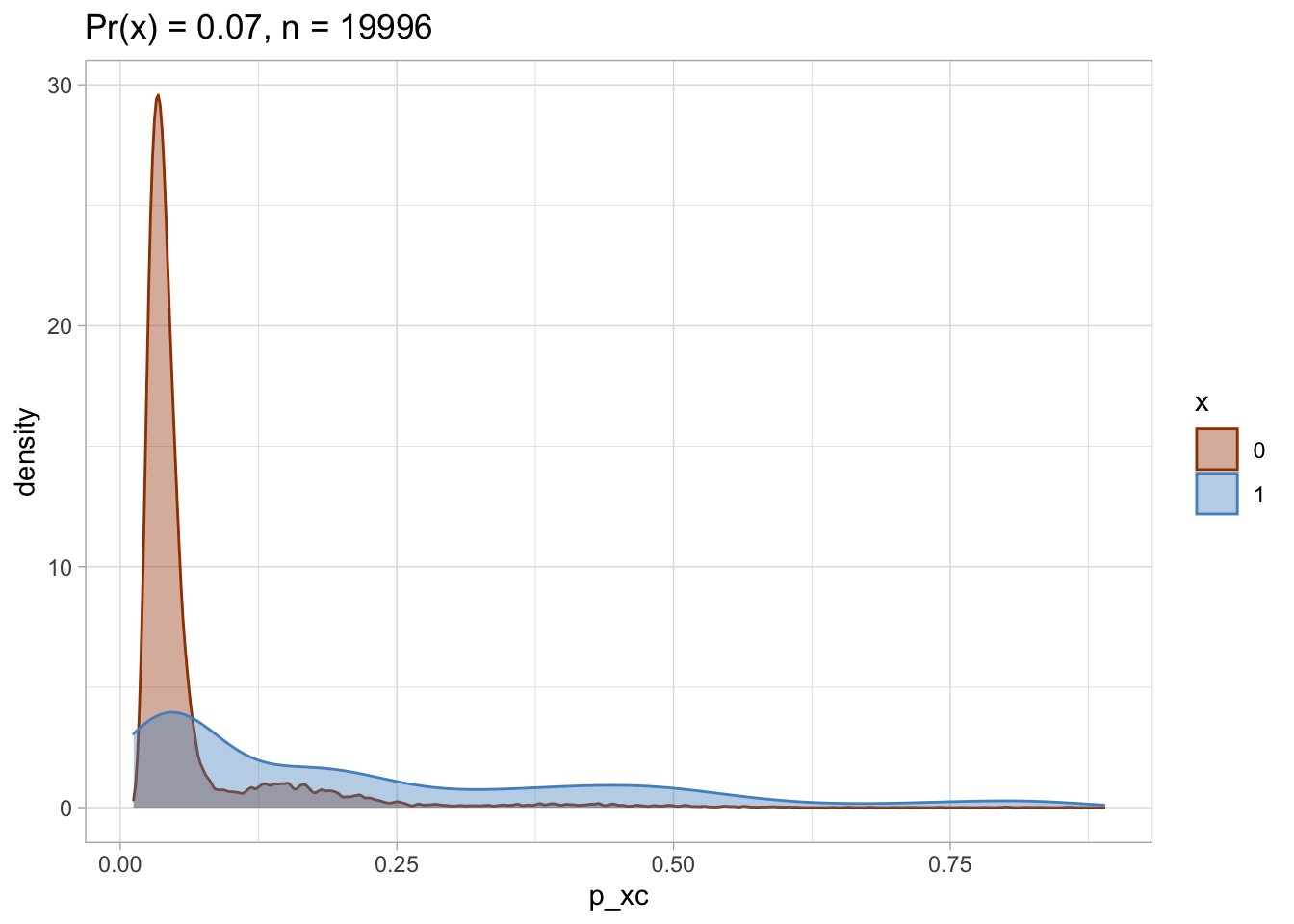##
## []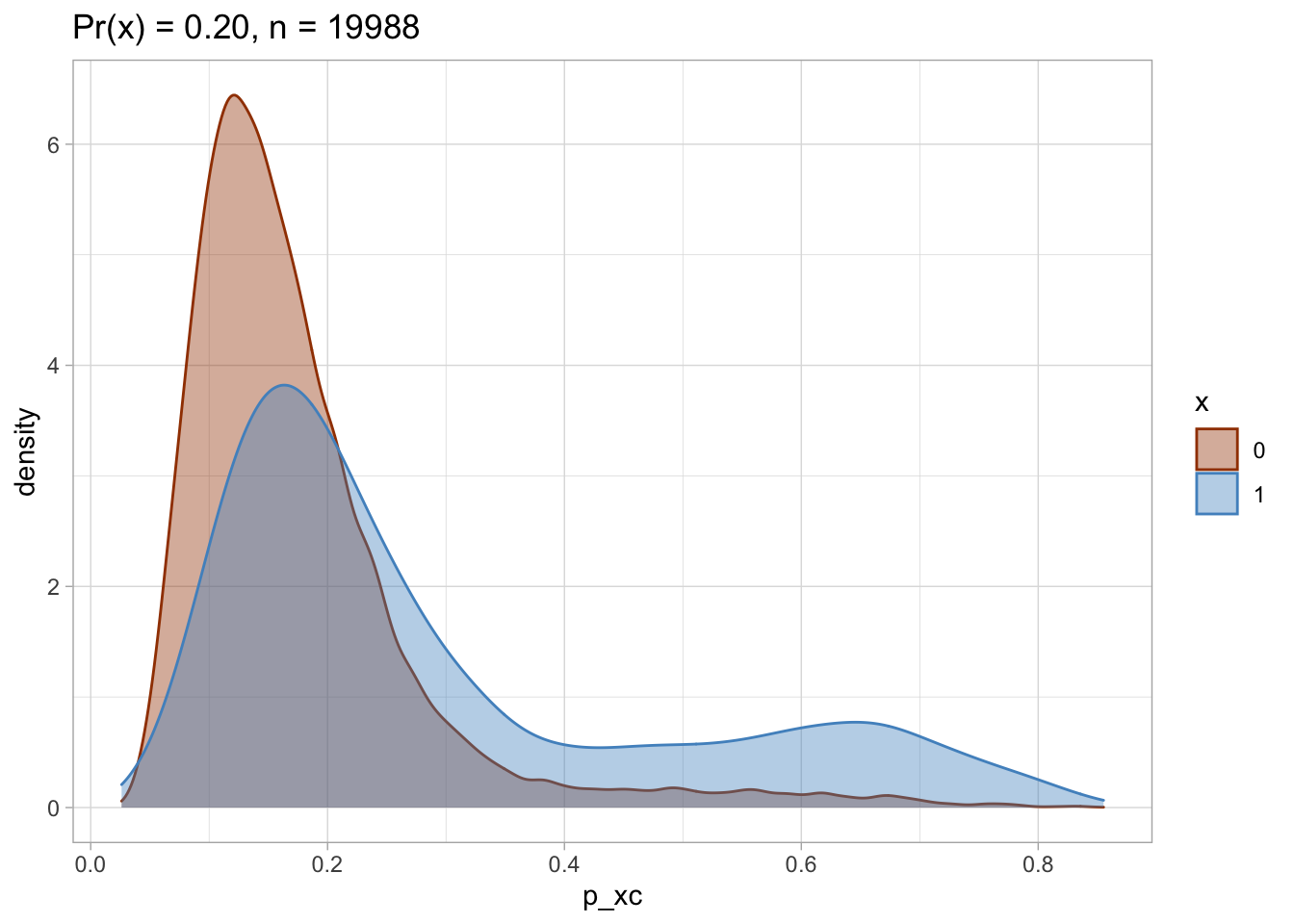nested\$viz_histo_trimmed

## []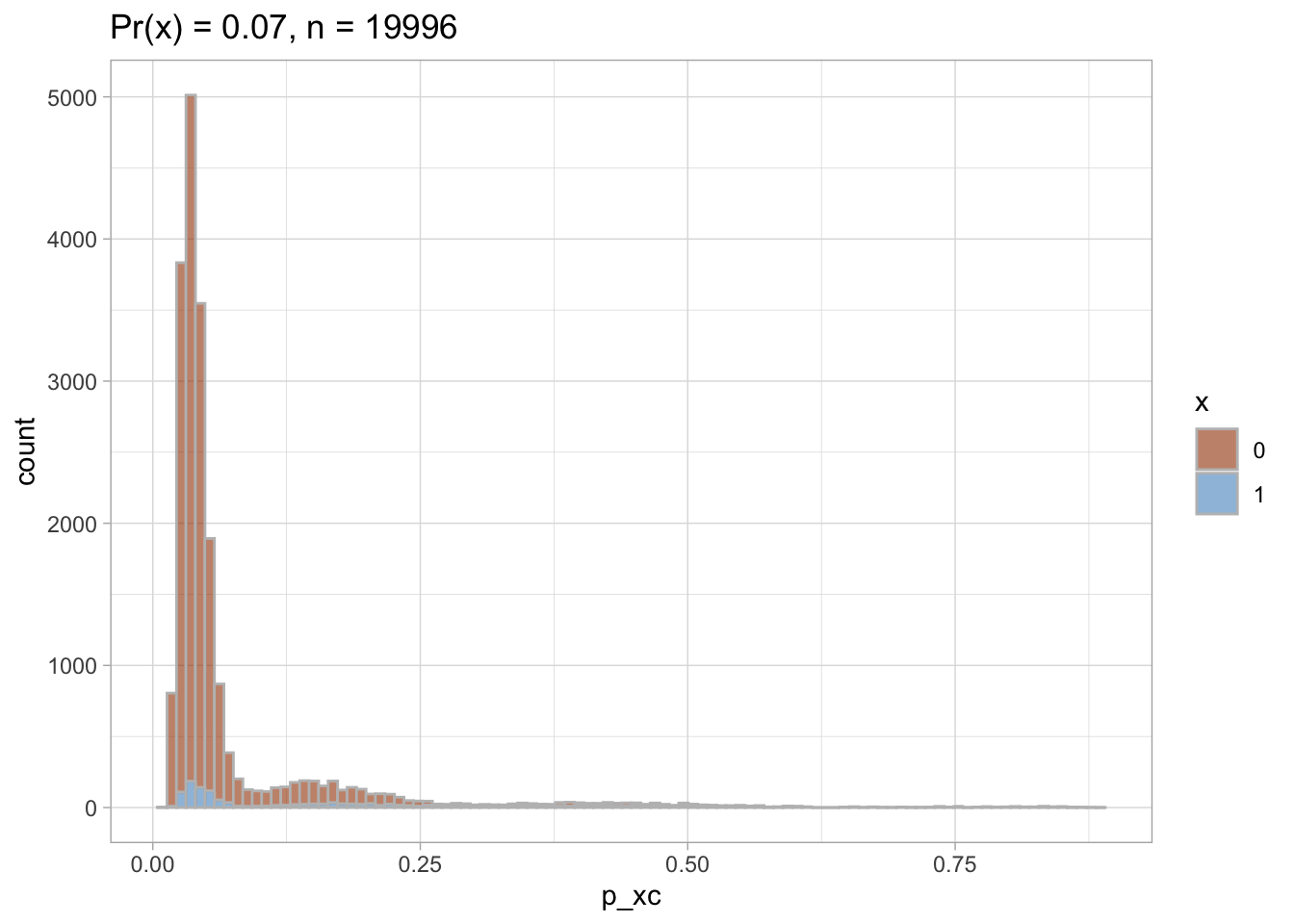##
## []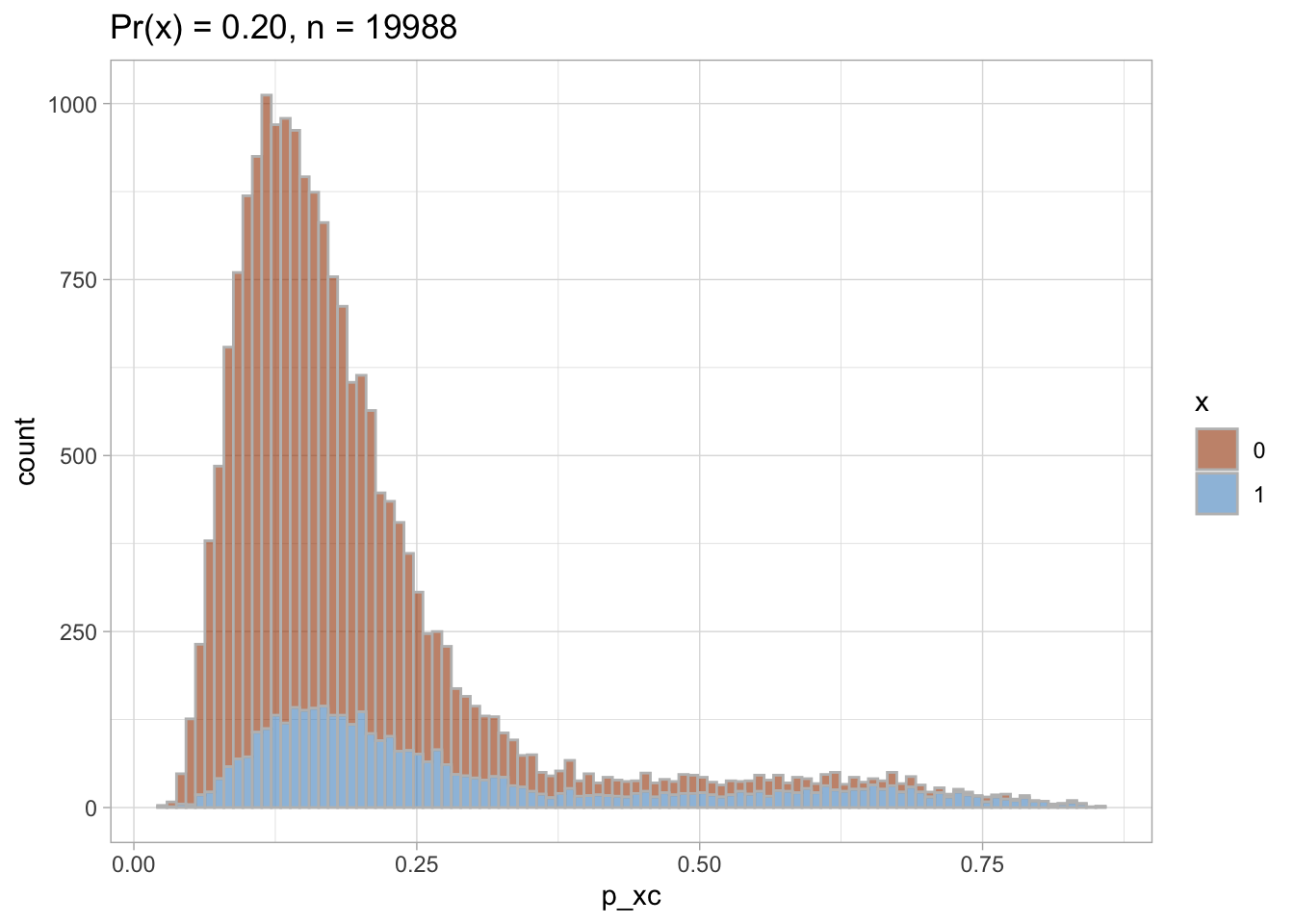### Standardized mortality ratio (SMR) weights I compute the standardized mortality ratio (SMR) weights to later use them for re-weighting of the unexposed as if they were exposed.
nested %<>% mutate(
ps = map(data_trimmed, ~ ps_model(.x)),
data_trimmed = map2(ps, data_trimmed, ~mutate(.y,
p_xc = predict(.x, type = "response"))),
data_trimmed = map(.x = data_trimmed,  ~mutate(.x,
# SMR weighting: the unexposed patients within the cohort receive weights equal to the ratio of (PS/(1 − PS))
smr.w = case_when(
x == 1 ~ 1, # weight in the exposed is 1
TRUE ~ (p_xc)/(1 - p_xc) # weight in the unexposed
)))
)

# check weights distribution
smr.w_distr <- nested %>%
select(data_trimmed, dataset) %>%
mutate(data_trimmed = map(data_trimmed, ~group_by(.x, x) %>%
summarise(min = min(smr.w),
mean = mean(smr.w),
max = max(smr.w),
p25 = quantile(smr.w, probs = 0.25),
p50 = quantile(smr.w, probs = 0.5),
p75 = quantile(smr.w, probs = 0.75)
))) %>%
unnest_legacy()

smr.w_distr %>% kableExtra::kable()

dataset x min mean max p25 p50 p75
Pr(x) = 0.07 0 0.0123614 0.0815847 7.995338 0.0321953 0.0413382 0.0563997
Pr(x) = 0.07 1 1.0000000 1.0000000 1.000000 1.0000000 1.0000000 1.0000000
Pr(x) = 0.20 0 0.0283421 0.2510004 5.832439 0.1265022 0.1787239 0.2615828
Pr(x) = 0.20 1 1.0000000 1.0000000 1.000000 1.0000000 1.0000000 1.0000000

## Results

For simplicity, I drop the bootstrapping for 95% CIs computation in the next part of the analyses. I compare how the results of different modeling approaches vary and whether they are able to close to the true null effect I simulated.

# CIs are not bootstrapped
nested %<>% mutate(
# crude
fit_crude_y1 = map(data, ~glm(data = .x, family = binomial(link = "logit"), formula = y1 ~ x)),
fit_crude_y1_tidy = map(fit_crude_y1, ~broom::tidy(., exponentiate = TRUE, conf.int = TRUE)),
fit_crude_y2 = map(data, ~glm(data = .x, family = binomial(link = "logit"), formula = y2 ~ x)),
fit_crude_y2_tidy = map(fit_crude_y2, ~broom::tidy(., exponentiate = TRUE, conf.int = TRUE)),
# SMR
fit_smr.w_y1 = map(data_trimmed, ~glm(data = .x, family = binomial(link = "logit"), formula = y1 ~ x, weights = smr.w)),
fit_smr.w_y1_tidy = map(fit_smr.w_y1, ~broom::tidy(., exponentiate = TRUE, conf.int = TRUE)),
fit_smr.w_y2 = map(data_trimmed, ~glm(data = .x, family = binomial(link = "logit"), formula = y2 ~ x, weights = smr.w)),
fit_smr.w_y2_tidy = map(fit_smr.w_y2, ~broom::tidy(., exponentiate = TRUE, conf.int = TRUE)),
fit_aor_y1 = map(data_trimmed, ~glm(data = .x, family = binomial(link = "logit"), formula = y1 ~ x + c1 + c2 + c3 + c4 + c5 + c6 + c7 + c8 + c9 + c10 + c13 + c14)),
fit_aor_y1_tidy = map(fit_aor_y1, ~broom::tidy(., exponentiate = TRUE, conf.int = TRUE)),
fit_aor_y2 = map(data_trimmed, ~glm(data = .x, family = binomial(link = "logit"), formula = y2 ~ x + c1 + c2 + c3 + c4 + c5 + c6 + c7 + c8 + c9 + c10 + c13 + c14)),
fit_aor_y2_tidy = map(fit_aor_y2, ~broom::tidy(., exponentiate = TRUE, conf.int = TRUE))
)


I extract the results of all models and visualize them using preferred colors.

results_1 <- nested %>%
select(dataset, prevalence_exposure, fit_crude_y1_tidy, fit_crude_y2_tidy, fit_smr.w_y1_tidy, fit_smr.w_y2_tidy, fit_aor_y1_tidy, fit_aor_y2_tidy) %>%
pivot_longer(c(3:8), names_to = "model", values_to = "results") %>%
unnest(cols = c(results)) %>%
filter(term == "x1") %>%
select(-p.value) %>%
separate(col = model, into = c("fit", "approach", "outcome", "tidy"), sep = "_", remove = T) %>%
select(-fit, -tidy) %>%
# pct bias on OR scale
# CLR: confidence limit ratio
mutate(
pct_bias = (estimate - 1) * 100,
clr = conf.high/conf.low,
analysis = row_number()
)

results_1 %>%
mutate(
approach = case_when(approach == "aor" ~ "Conventional adjustment",
approach == "crude" ~ "Crude",
approach == "smr.w" ~ "SMR weights"),
approach = factor(approach, levels = rev(c("SMR weights", "Conventional adjustment", "Crude")))
) %>%
ggplot(aes(x = as_factor(prevalence_exposure), y = estimate, color = outcome, fill = outcome, shape = outcome)) +
geom_hline(yintercept = 1, color = "gray") +
geom_errorbar(aes(ymin=conf.low, ymax = conf.high), width = 0.2, position = position_dodge(width = 0.3)) +
geom_point(position = position_dodge(width = 0.3)) +
coord_flip() +
facet_grid(rows = vars(approach)) +
theme_light() +
scale_color_manual(values = palette_ps[c(10, 9, 4)]) +
scale_fill_manual(values = palette_ps[c(10, 9, 4)]) +
labs(x = "Exposure prevalence", y = "OR 95% CI") +
guides(fill=guide_legend(title = "Outcome"), color = guide_legend(title = "Outcome"), shape = guide_legend(title = "Outcome"))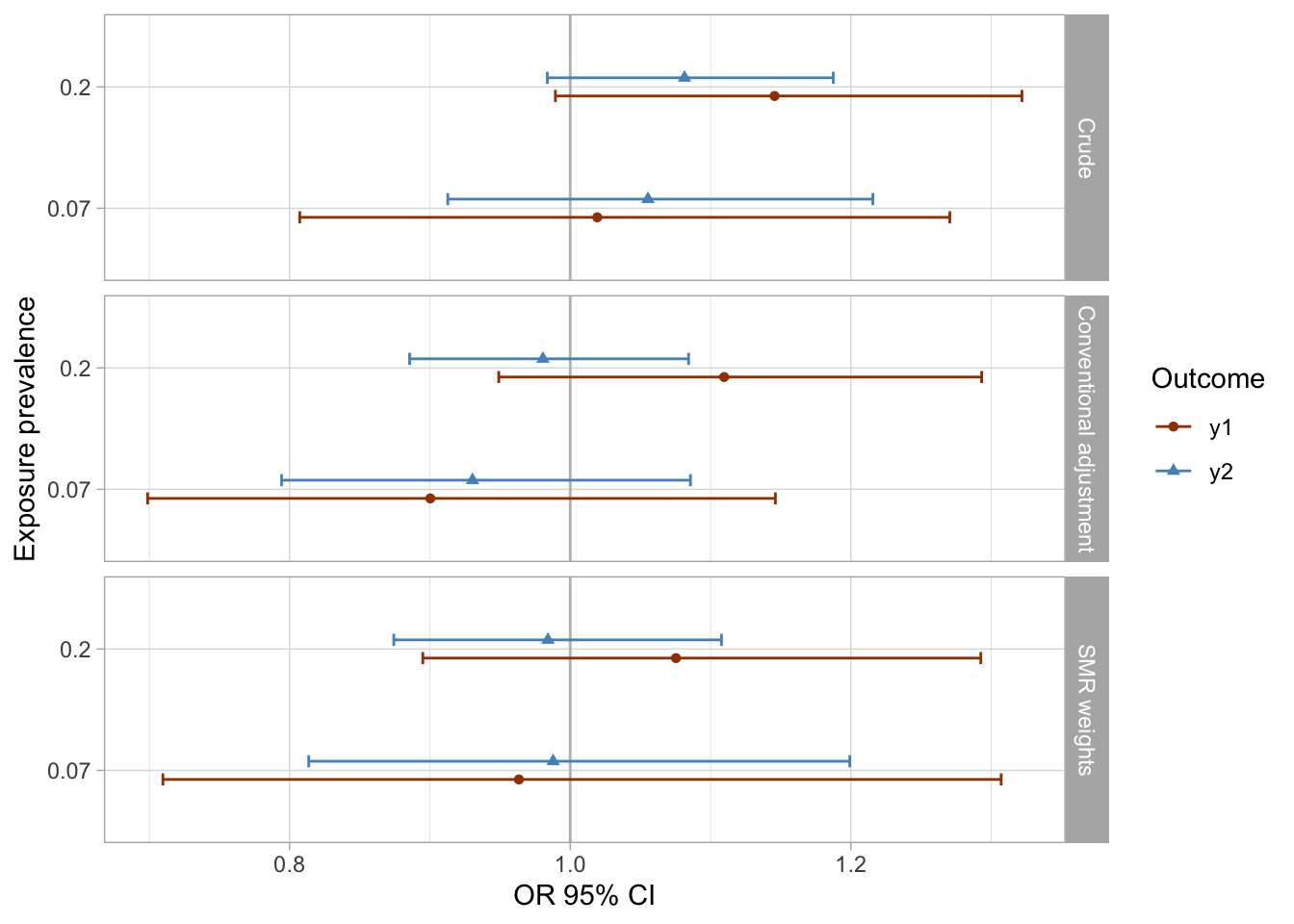results_1 %>%
mutate(
approach = case_when(approach == "aor" ~ "Conventional adjustment",
approach == "crude" ~ "Crude",
approach == "smr.w" ~ "SMR weights"),
approach = factor(approach, levels = rev(c("SMR weights", "Conventional adjustment", "Crude")))
) %>%
ggplot(aes(x = as_factor(prevalence_exposure), y = pct_bias, color = outcome, fill = outcome, shape = outcome, group = outcome)) +
geom_hline(yintercept = 0, color = "gray") +
geom_point() +
facet_grid(rows = vars(approach)) +
theme_light() +
coord_flip() +
scale_y_continuous(limits = c(-20, 20), breaks = seq(-20, 20, 5)) +
scale_color_manual(values = palette_ps[c(10, 9)]) +
scale_fill_manual(values = palette_ps[c(10, 9)])+
labs(x = "Exposure prevalence", y = "% bias") +
guides(fill=guide_legend(title = "Outcome"), color = guide_legend(title = "Outcome"), shape = guide_legend(title = "Outcome"))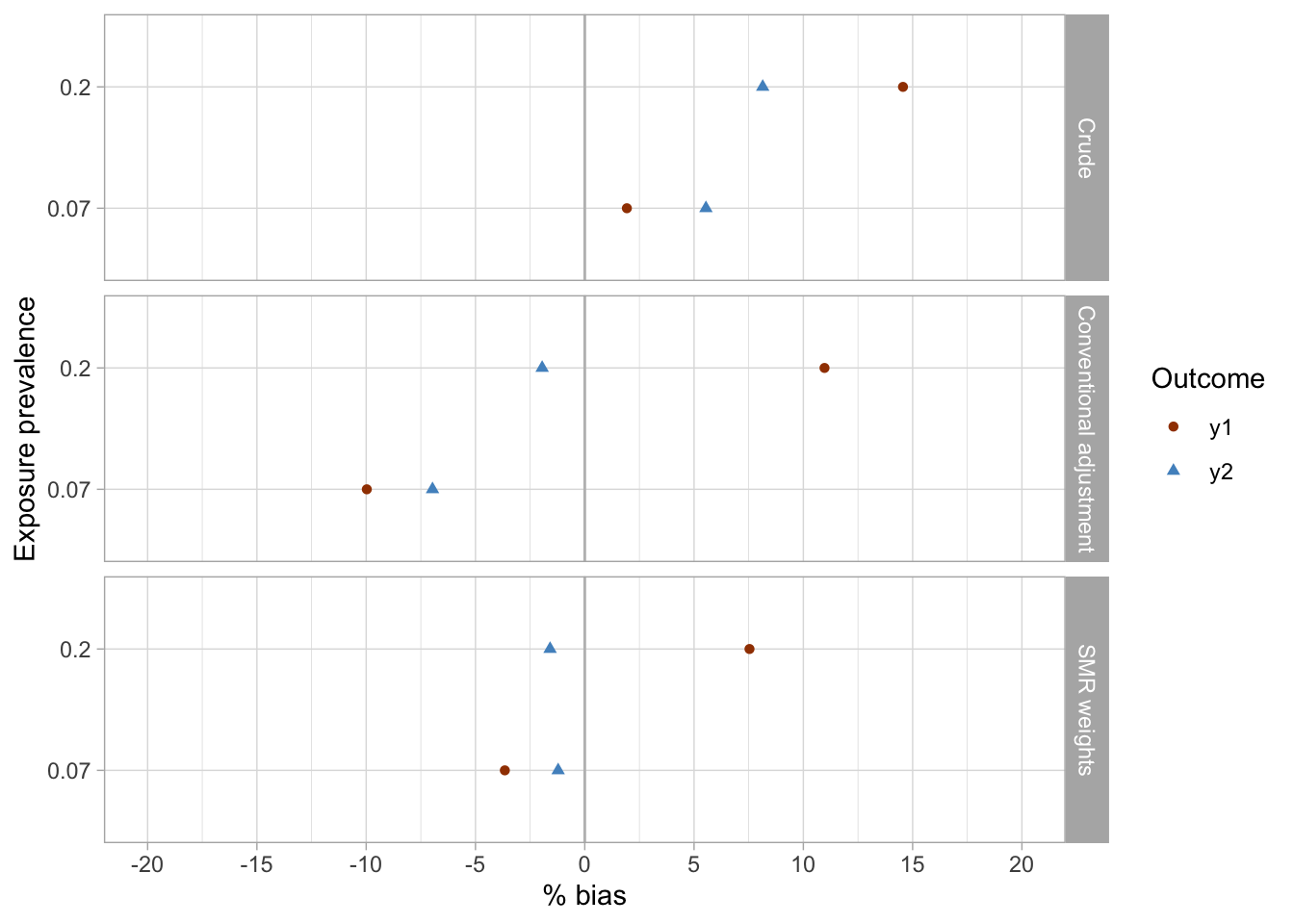## Conclusions

• The crude analyses were imprecise and suggested associations were present between the exposures (x1, x2) and the outcomes (y1, y2), while there were null true associations (maximum bias in crude analyses was approximately 15%).
• The conventional adjustment in the logistic regression model produced imprecise estimates and suggested weak associations, including reverse associations for some exposure-outcome pairs (maximum bias of approximately 11%).
• SMR weighting produced imprecise results and similarly to conventional adjustment suggested some weak inverse associations between exposures and outcomes (maximum bias of approximately 7.5% was observed for the x2-y1 exposure-outcome pair).
• Although uncertainty presented an issue in all three analyses, the SMR weighting approach arguably produced the point estimates closest to the true (simulated) null effects.
1. Desai RJ, Rothman KJ, Bateman BT, Hernandez-Diaz S, Huybrechts KF. A Propensity-score-based Fine Stratification Approach for Confounding Adjustment When Exposure Is Infrequent. Epidemiology. 2017;28(2):249-257. doi:10.1097/EDE.0000000000000595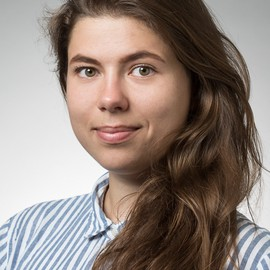##### Elena Dudukina
###### Clinical Specialist and PhD student

I am interested in women’s health, reproductive epidemiology, pharmacoepidemiology, causal inference, directed acyclic graphs, and R stats.